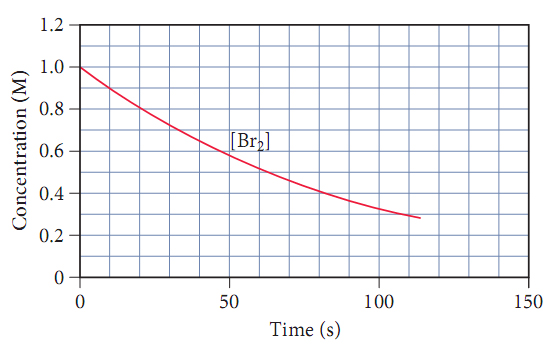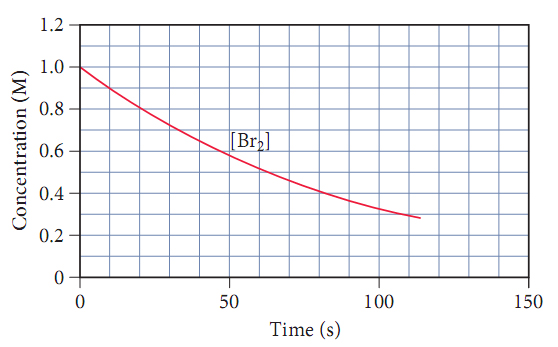# Problem: Consider the following reaction: H2(g) + Br2(g) → 2HBr. The graph below shows the concentration of Br2 as a function of time.Use the graph to calculate the instantaneous rate of the reaction at 25 s.

###### FREE Expert Solution

We’re being asked to calculate the instantaneous rate of the reaction at 25 s based on the graph given.An instantaneous reaction rate is the rate at a particular moment in the reaction and is usually determined graphically and can be calculated as:

Δ[  ] = change in concentration
Δt = change in time

91% (389 ratings)###### Problem Details

Consider the following reaction: H2(g) + Br2(g) → 2HBr. The graph below shows the concentration of Br2 as a function of time.Use the graph to calculate the instantaneous rate of the reaction at 25 s.

Frequently Asked Questions

What scientific concept do you need to know in order to solve this problem?

Our tutors have indicated that to solve this problem you will need to apply the Instantaneous Rate of Change concept. If you need more Instantaneous Rate of Change practice, you can also practice Instantaneous Rate of Change practice problems.

What professor is this problem relevant for?

Based on our data, we think this problem is relevant for Professor Lin's class at CU.